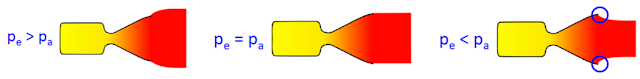### Section 3.3 - Rocket Thrust

#### The Rocket Thrust Equation

The thrust produced by a rocket can be calculated with the following equation:

$T = {\dot m}V_e + (p_e - p_a)A_e$

$T =$ thrust
${\dot m} =$mass flow rate
$V_e =$exhaust velocity
$p_e =$ exhaust pressure at nozzle exit
$p_a =$ ambient pressure at nozzle exit
$A_e =$nozzle exit area

You'll notice that the right side of the equals sign has two terms:   ${\dot m}V_e$   and   $(p_e - p_a)A_e$. Basically what it's telling us is that thrust is generated by two factors

1. The momentum generated by the exhaust leaving the nozzle (which is much larger in magnitude)
2. The pressure difference between the exhaust and the atmospheric pressure across the area of the nozzle. Think of this as the natural diffusion of a gas from a region of high pressure to low pressure, slightly adding to thrust

#### Example

A rocket engine ejects mass at a rate of 30 kg/s with an exhaust velocity of 3,100 m/s. The exhaust pressure is 5kPa and the exit area is 0.7$m^2$. What is the thrust of the engine in a vacuum?

a) 9,650 N
b) 19,300 N
c) 48,250 N
d) 96,500 N

Solution: d

$T = 30 \cdot 3100 + (5000 - 0) \cdot 0.7$

Recall that a Pascal is a unit of pressure defined as the force of 1 Newton over an area of 1$m^2$. Additionally, the problem tells us the engine is exhausting into a vacuum, so $p_a = 0$

#### Optimal Exhaust Pressure

From the rocket thrust equation, we might be tempted to make $p_e$ as large as possible relative to $p_a$ to squeeze out some added thrust. In reality, engineers want exhaust pressure to equal ambient pressure ($p_e = p_a$) for optimal efficiency. Why? Let's consider the options:Left: underexpanded | Center: Optimized | Right: Overexpanded

• Underexpanded nozzle ($p_e > p_a$): this nozzle is not as efficient as it could be because some of the exhaust is being directed at an angle instead of straight downward
• Overexpanded nozzle ($p_e < p_a$): this nozzle is dangerous because flow separation can occur at the circled areas and cause damaging vibrations which can cause structural failure

So we've decided to try set $p_e = p_a$. Here's the problem: ambient pressure is constantly changing (pressure decreases as the rocket achieves higher and higher altitudes) so there's no way to always match $p_a$. The best we can do is compromise at a middle ground - have the rocket nozzle be slightly overexpanded at sea level, and as it rises it'll become more and more underexpanded and lose efficiency. Rocket staging can also help solve this problem, as each stage has its own set of rocket engines that can be optimized for different altitudes (more on staging later)

For more on rocket engine design and other rocket science topics, this website is by far the best I've found for non-engineers who still want some technical understanding: http://www.braeunig.us/space/. More technical than what I have here but definitely worth a shot!